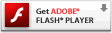# Interactive Generative Art Series – 01 – ColorAfter updating the line width to be still random, but more of a gradual step in variation (in the first experiment in this series), the second most obvious edit to the original in this generative art series is the color of the line. While it would be pretty simple to update the code to use any one solid color in place of the black, I wanted the color to vary over time. The simplest way I know to achieve this is to create a variable to hold the color value (as a number) and then change it over time. So here, I have a color chosen at random and just increment it every time the loop function executes by 1024. I chose this amount because it would loop through and eventually get back to where it started while restricting the color scheme. I think it brings a lot to the design to have color – and I especially like how it randomly creates a color scheme and sticks to it. Totally random colors may look a bit much, while problematically it’s not too difficult to get, it may be difficult to look at once it’s created. Above are a few screen shots of the random colors generated:

### 01 Color, play here

Please visit the blog article to view this interactive flash content. Flash plug-in required:### Source Code

[cc lang=”actionscript”]
var ball:Sprite = new Sprite();

ball.graphics.beginFill(0x333333, 1);
ball.graphics.drawCircle(0, 0, 30);
ball.graphics.endFill();

var div:Number = .1;

var ax:Number = 0;
var ay:Number = 0;

var line_max_width:Number = 50;
var line_min_width:Number = 0;
var line_width:Number = Math.random() * line_max_width;
var line_width_velocity:Number = 0;
var dampen:Number = 0.95;

var color:Number = Math.floor(Math.random() * 16777215);

function loop () {
ball.x -= ax = (ax + (ball.x – mouseX) * div) * .9;
ball.y -= ay = (ay + (ball.y – mouseY) * div) * .9;

line_width_velocity += Math.random() * 6 – 3;
line_width += line_width_velocity;
line_width_velocity *= dampen;
if(line_width > line_max_width) { line_width = line_max_width; }
if (line_width < line_min_width) { line_width = line_min_width; }
this.graphics.lineStyle(line_width, color+=1024, 1);
this.graphics.lineTo(ball.x, ball.y);
}

setInterval(loop, 1000/30);
[/cc]
You’ll see if you’re following along that this only add 2 lines of code from the last version. We simply create and instantiate (with a random value) the color variable and then apply it in place of the black to the lineStyle and simultaneously increment it. Check the example swf here and get the fla here.